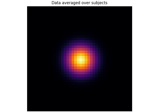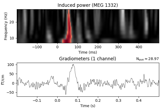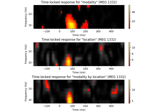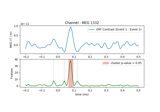# mne.stats.permutation_cluster_test#

mne.stats.permutation_cluster_test(X, threshold=None, n_permutations=1024, tail=0, stat_fun=None, adjacency=None, n_jobs=None, seed=None, max_step=1, exclude=None, step_down_p=0, t_power=1, out_type='indices', check_disjoint=False, buffer_size=1000, verbose=None)[source]#

Cluster-level statistical permutation test.

For a list of `NumPy arrays` of data, calculate some statistics corrected for multiple comparisons using permutations and cluster-level correction. Each element of the list `X` should contain the data for one group of observations (e.g., 2D arrays for time series, 3D arrays for time-frequency power values). Permutations are generated with random partitions of the data. For details, see .

Parameters:
X`list` of `array`, shape (n_observations, p[, q][, r])

The data to be clustered. Each array in `X` should contain the observations for one group. The first dimension of each array is the number of observations from that group; remaining dimensions comprise the size of a single observation. For example if `X = [X1, X2]` with `X1.shape = (20, 50, 4)` and `X2.shape = (17, 50, 4)`, then `X` has 2 groups with respectively 20 and 17 observations in each, and each data point is of shape `(50, 4)`. Note: that the last dimension of each element of `X` should correspond to the dimension represented in the `adjacency` parameter (e.g., spectral data should be provided as `(observations, frequencies, channels/vertices)`).

threshold

The so-called “cluster forming threshold” in the form of a test statistic (note: this is not an alpha level / “p-value”). If numeric, vertices with data values more extreme than `threshold` will be used to form clusters. If `None`, an F-threshold will be chosen automatically that corresponds to a p-value of 0.05 for the given number of observations (only valid when using an F-statistic). If `threshold` is a `dict` (with keys `'start'` and `'step'`) then threshold-free cluster enhancement (TFCE) will be used (see the TFCE example and ). See Notes for an example on how to compute a threshold based on a particular p-value for one-tailed or two-tailed tests.

n_permutations`int`

The number of permutations to compute.

tail`int`

If tail is 1, the statistic is thresholded above threshold. If tail is -1, the statistic is thresholded below threshold. If tail is 0, the statistic is thresholded on both sides of the distribution.

stat_fun

Function called to calculate the test statistic. Must accept 1D-array as input and return a 1D array. If `None` (the default), uses `mne.stats.f_oneway`.

Defines adjacency between locations in the data, where “locations” can be spatial vertices, frequency bins, time points, etc. For spatial vertices, see: `mne.channels.find_ch_adjacency()`. If `False`, assumes no adjacency (each location is treated as independent and unconnected). If `None`, a regular lattice adjacency is assumed, connecting each location to its neighbor(s) along the last dimension of each group `X[k]` (or the last two dimensions if `X[k]` is 2D). If `adjacency` is a matrix, it is assumed to be symmetric (only the upper triangular half is used) and must be square with dimension equal to `X[k].shape[-1]` (for 2D data) or `X[k].shape[-1] * X[k].shape[-2]` (for 3D data) or (optionally) `X[k].shape[-1] * X[k].shape[-2] * X[k].shape[-3]` (for 4D data). The function `mne.stats.combine_adjacency` may be useful for 4D data.

n_jobs

The number of jobs to run in parallel. If `-1`, it is set to the number of CPU cores. Requires the `joblib` package. `None` (default) is a marker for ‘unset’ that will be interpreted as `n_jobs=1` (sequential execution) unless the call is performed under a `joblib.parallel_backend()` context manager that sets another value for `n_jobs`.

seed`None` | `int` | instance of `RandomState`

A seed for the NumPy random number generator (RNG). If `None` (default), the seed will be obtained from the operating system (see `RandomState` for details), meaning it will most likely produce different output every time this function or method is run. To achieve reproducible results, pass a value here to explicitly initialize the RNG with a defined state.

max_step`int`

Maximum distance between samples along the second axis of `X` to be considered adjacent (typically the second axis is the “time” dimension). Only used when `adjacency` has shape (n_vertices, n_vertices), that is, when adjacency is only specified for sensors (e.g., via `mne.channels.find_ch_adjacency()`), and not via sensors and further dimensions such as time points (e.g., via an additional call of `mne.stats.combine_adjacency()`).

exclude

Mask to apply to the data to exclude certain points from clustering (e.g., medial wall vertices). Should be the same shape as `X`. If `None`, no points are excluded.

step_down_p`float`

To perform a step-down-in-jumps test, pass a p-value for clusters to exclude from each successive iteration. Default is zero, perform no step-down test (since no clusters will be smaller than this value). Setting this to a reasonable value, e.g. 0.05, can increase sensitivity but costs computation time.

t_power`float`

Power to raise the statistical values (usually F-values) by before summing (sign will be retained). Note that `t_power=0` will give a count of locations in each cluster, `t_power=1` will weight each location by its statistical score.

Output format of clusters within a list. If `'mask'`, returns a list of boolean arrays, each with the same shape as the input data (or slices if the shape is 1D and adjacency is None), with `True` values indicating locations that are part of a cluster. If `'indices'`, returns a list of tuple of ndarray, where each ndarray contains the indices of locations that together form the given cluster along the given dimension. Note that for large datasets, `'indices'` may use far less memory than `'mask'`. Default is `'indices'`.

check_disjoint`bool`

Whether to check if the connectivity matrix can be separated into disjoint sets before clustering. This may lead to faster clustering, especially if the second dimension of `X` (usually the “time” dimension) is large.

buffer_size

Block size to use when computing test statistics. This can significantly reduce memory usage when `n_jobs > 1` and memory sharing between processes is enabled (see `mne.set_cache_dir()`), because `X` will be shared between processes and each process only needs to allocate space for a small block of locations at a time.

verbose

Control verbosity of the logging output. If `None`, use the default verbosity level. See the logging documentation and `mne.verbose()` for details. Should only be passed as a keyword argument.

Returns:
F_obs`array`, shape (p[, q][, r])

Statistic (F by default) observed for all variables.

clusters`list`

List type defined by out_type above.

cluster_pv`array`

P-value for each cluster.

H0`array`, shape (n_permutations,)

Max cluster level stats observed under permutation.

Notes

For computing a `threshold` based on a p-value, use the conversion from `scipy.stats.rv_continuous.ppf()`:

```pval = 0.001  # arbitrary
dfn = n_conditions - 1  # degrees of freedom numerator
dfd = n_observations - n_conditions  # degrees of freedom denominator
thresh = scipy.stats.f.ppf(1 - pval, dfn=dfn, dfd=dfd)  # F distribution
```

References

## Examples using `mne.stats.permutation_cluster_test`#Statistical inference

Statistical inferenceNon-parametric between conditions cluster statistic on single trial power

Non-parametric between conditions cluster statistic on single trial powerMass-univariate twoway repeated measures ANOVA on single trial power

Mass-univariate twoway repeated measures ANOVA on single trial powerPermutation F-test on sensor data with 1D cluster level

Permutation F-test on sensor data with 1D cluster level